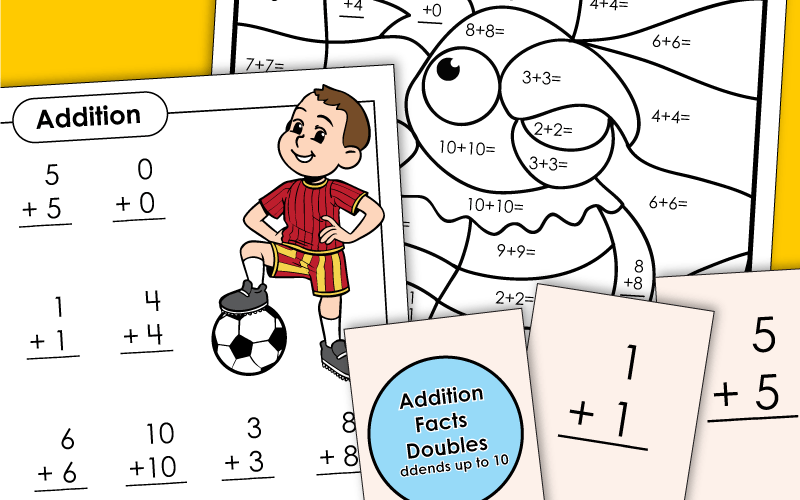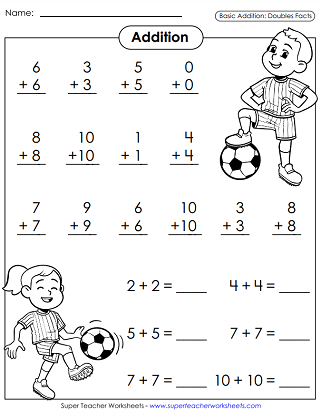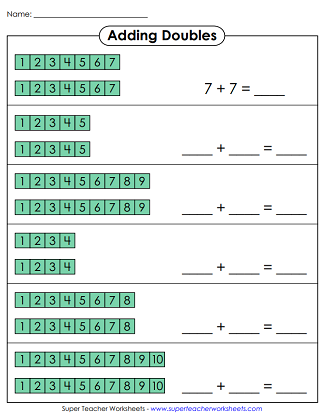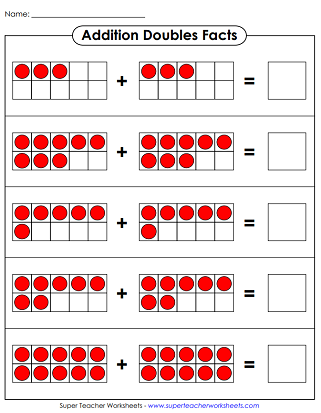An addition doubles fact is a math fact where the same number is added twice. (For example: 2+2=4; 5+5=10; 9+9=18) This page has printable worksheets and activities for teaching doubles facts.## Graphical Concept Worksheets: Addition Doubles Facts

Use the number block models to help find the answers to the basic addition facts.
Use red and white counters to find the answers to the doubles facts.
This worksheet has a pair of tens frames with an equal number of counters in each. Add up the number of counters in each to find the answer.
Students use the domino images to write out equations and add the doubles.

## Practice Worksheets

Quickly and accurately solve the addition basic facts. All facts are doubles, from 0+0 up through 10+10. Features illustrations of children playing soccer.
Work on improving speed and accuracy with this addition doubles drill worksheet. Features illustrations of children wearing animal masks.
Solve each word problem with an addition doubles fact.
Write the answers to the addition facts (doubles, up to 10+10). Then color according to the key. The finished picture is a whale.
Write the answer to each basic addition fact (doubles). Then use the color key at the bottom to complete the picture.

## Class Game / Learning CenterAddition Doubles Facts

In this memory match card game, students take turns trying to match addition facts with their sums. Makes a great game for small groups or learning center activity. (Recommendation: Print on card stock so students cannot see through the cards.)

## Flashcards and Sliders

Use these flash cards to practice adding doubles up to 10.
Weave the math fact strip into the pirate ship. Slide it up and down to reveal doubles facts. (For best results, print on thick card stock paper.)

Super Teacher has hundreds of worksheets for teaching basic addition concepts and fact fluency.

## Sample Worksheet ImagesMy Account
Site Information